Anúncio

# My c++

snathick
8 de Aug de 2011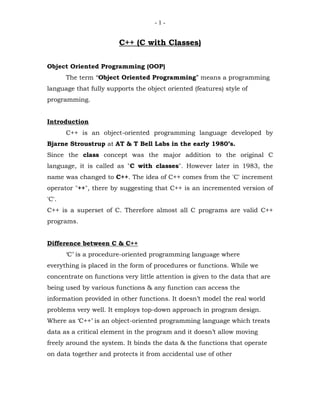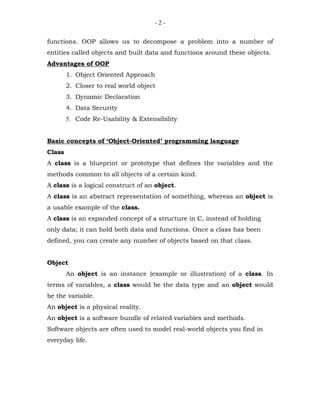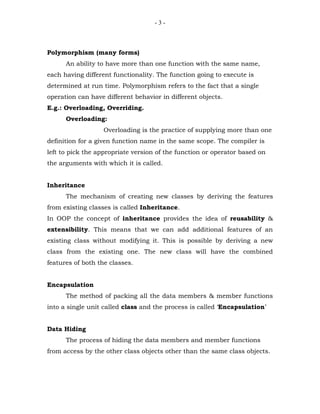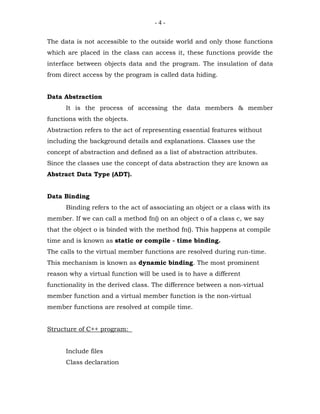Anúncio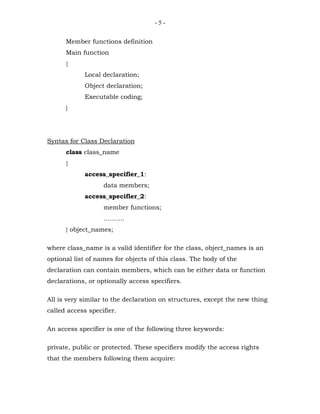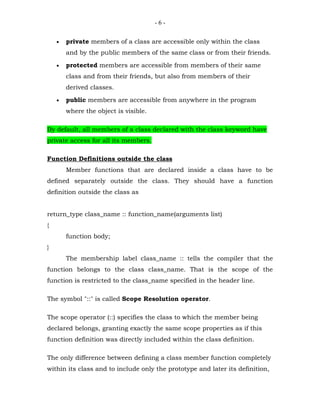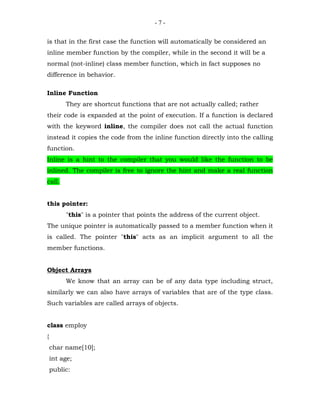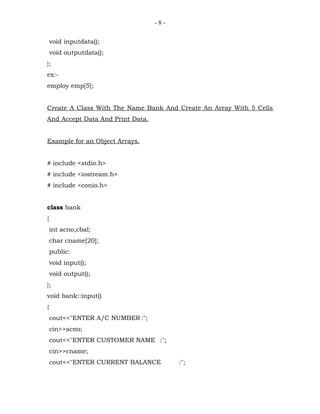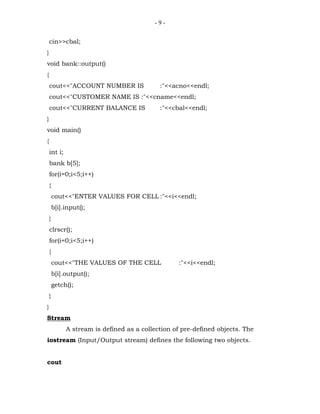Anúncio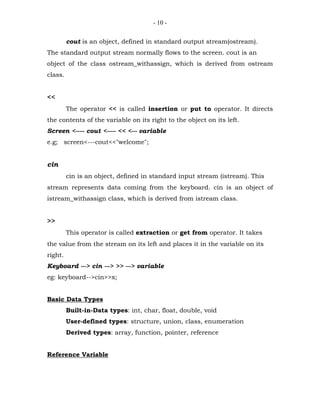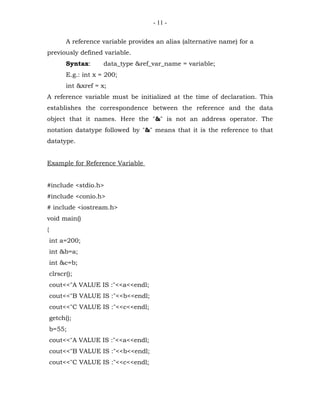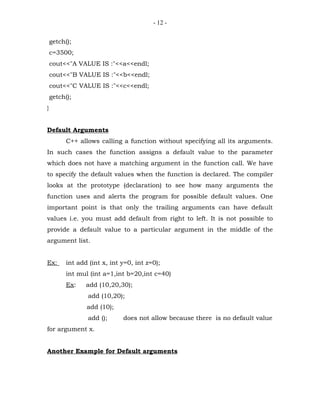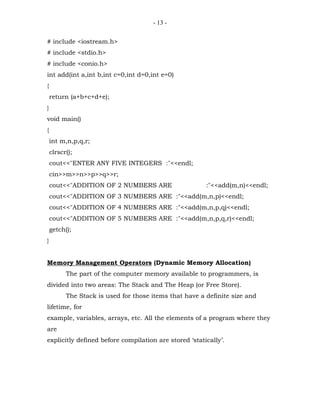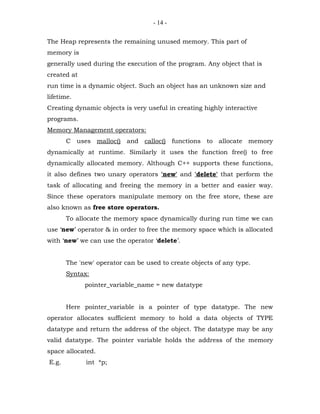Anúncio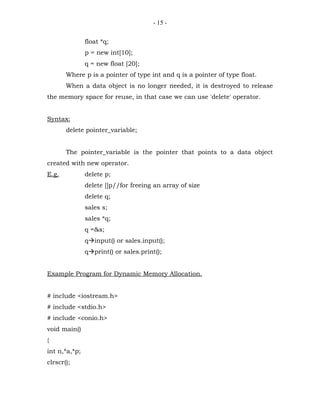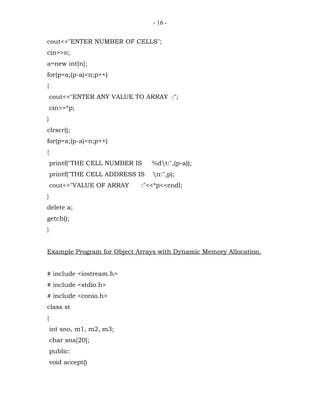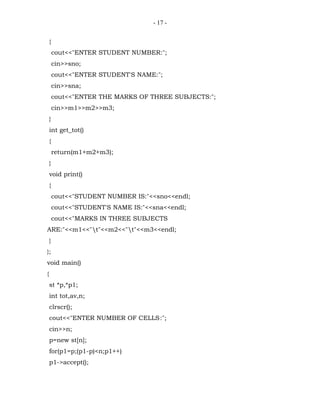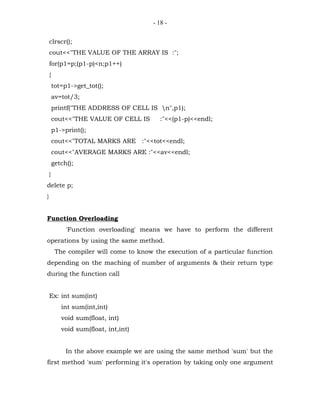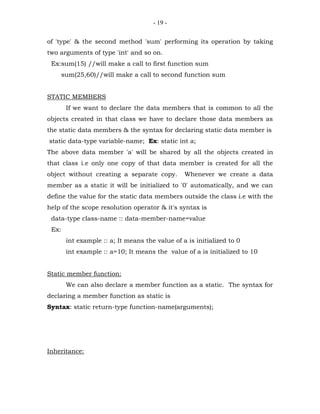Anúncio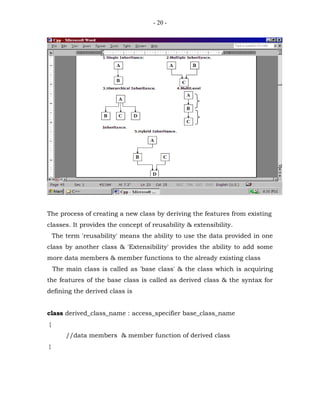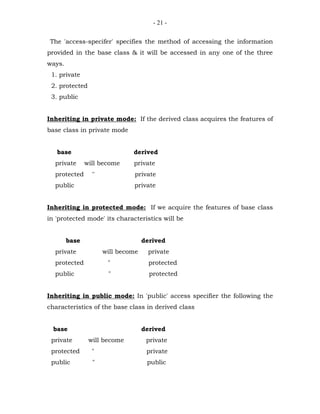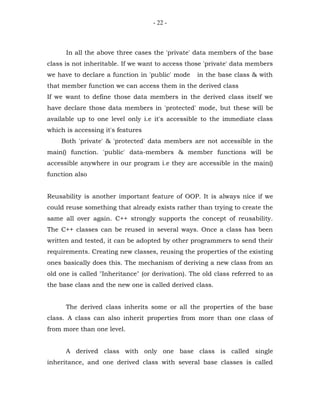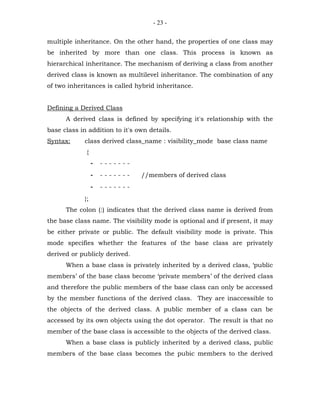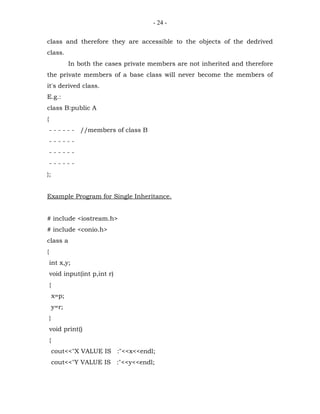Anúncio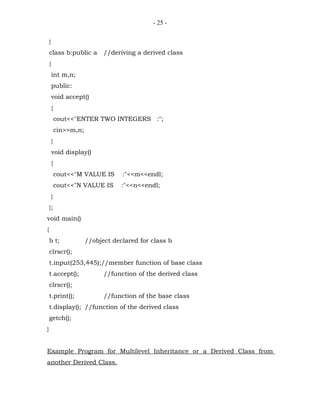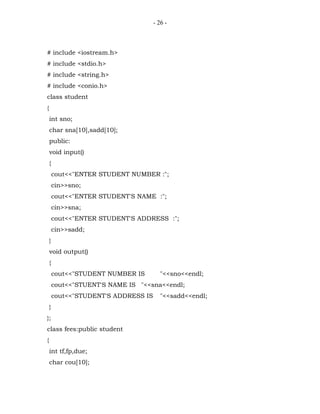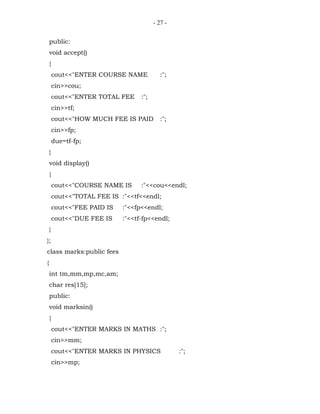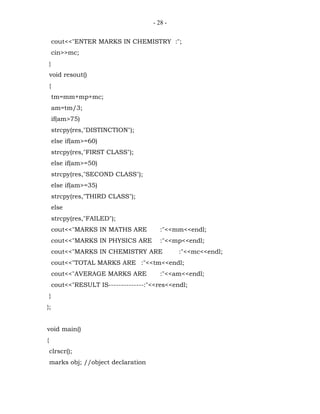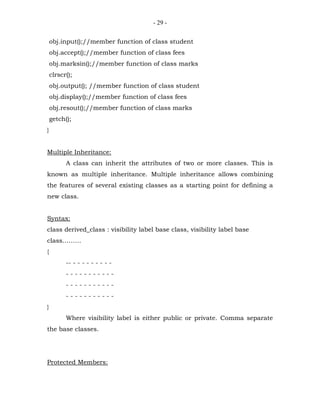Anúncio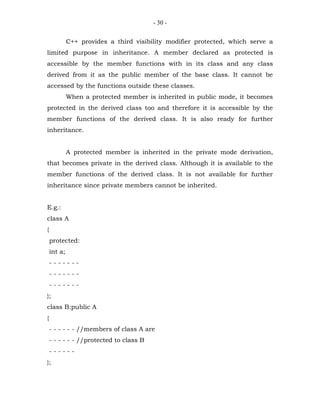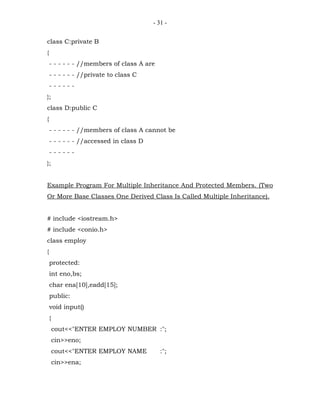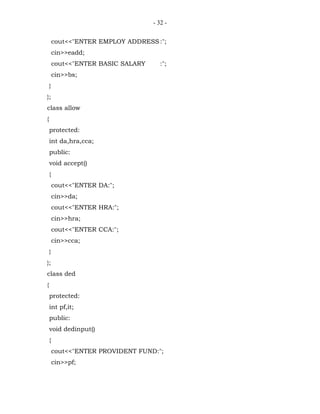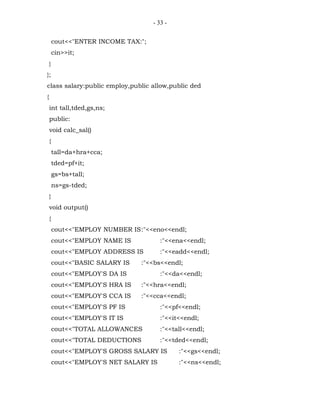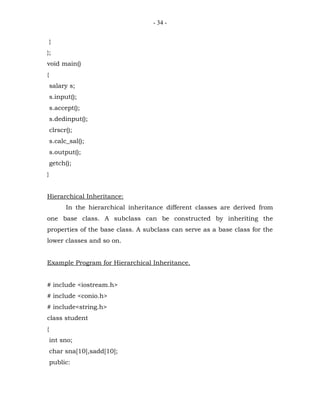Anúncio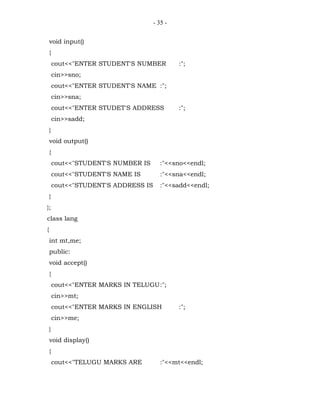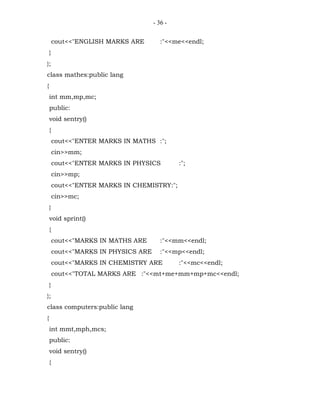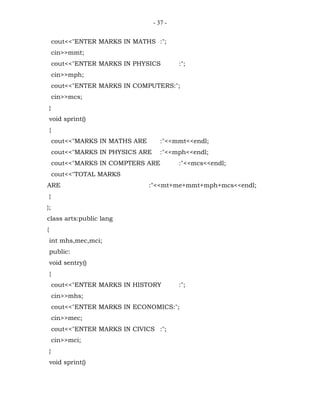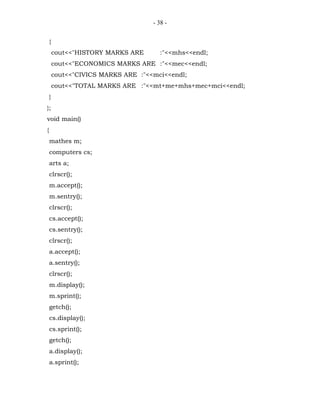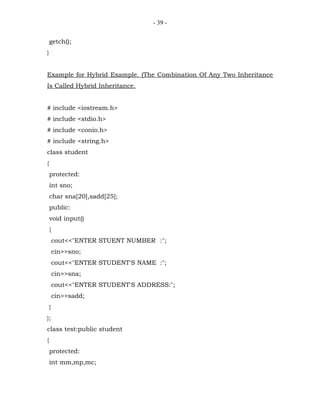Anúncio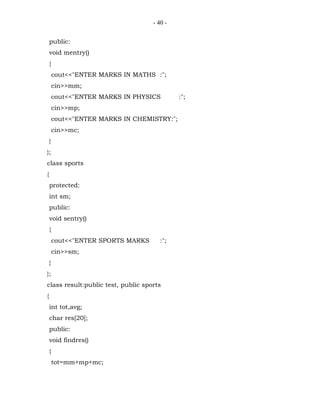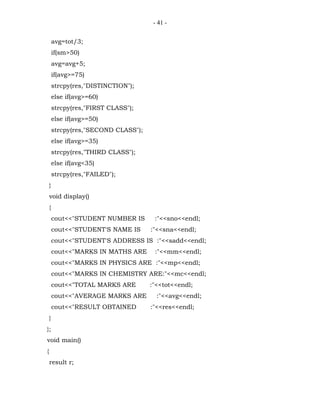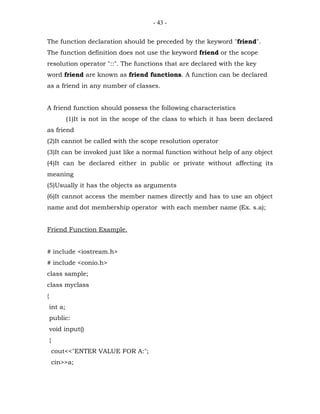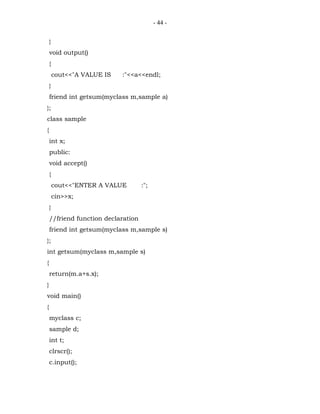Anúncio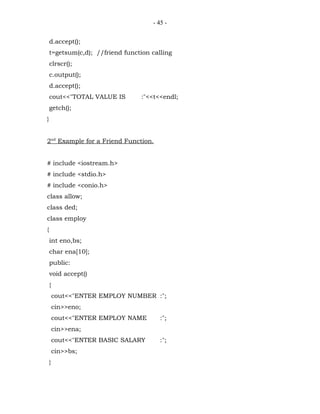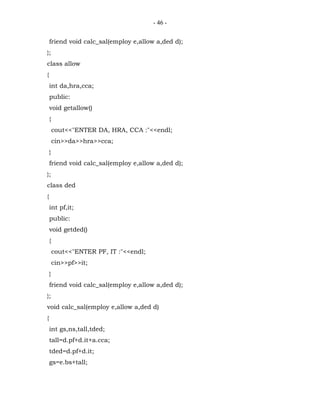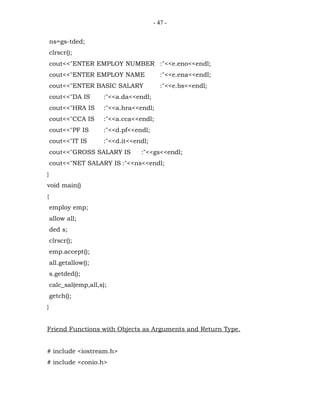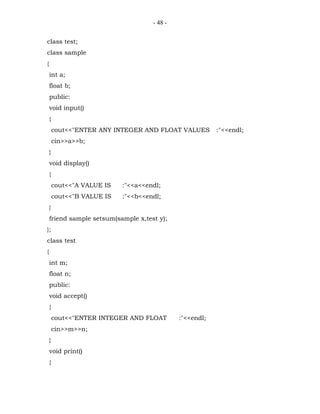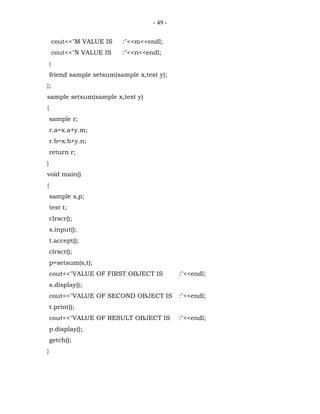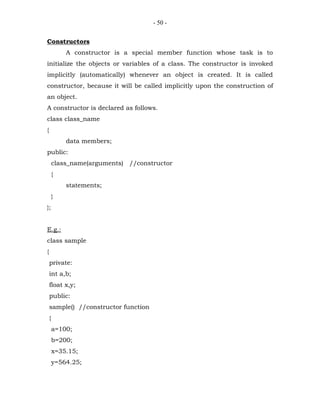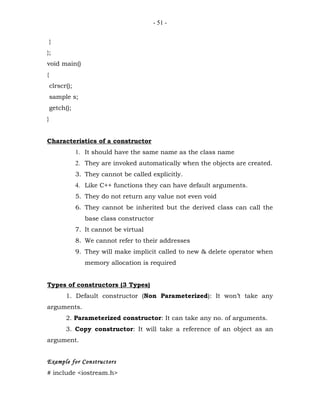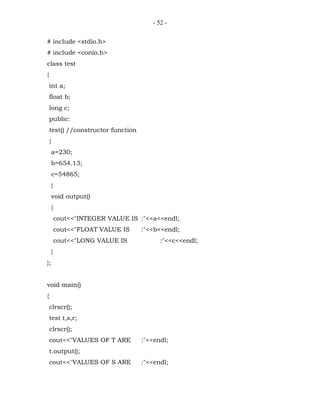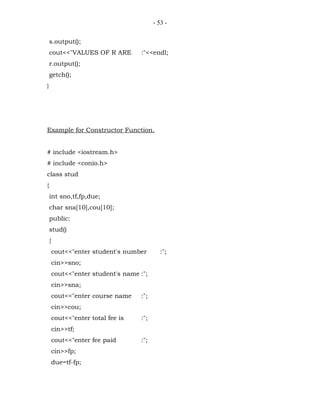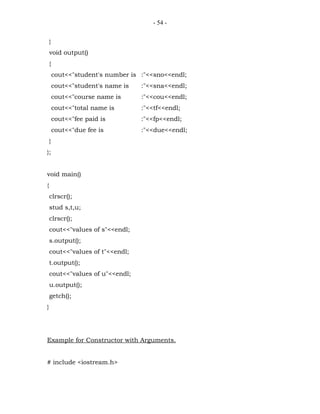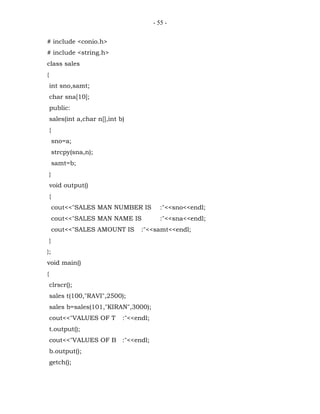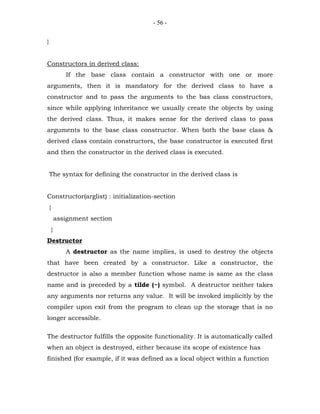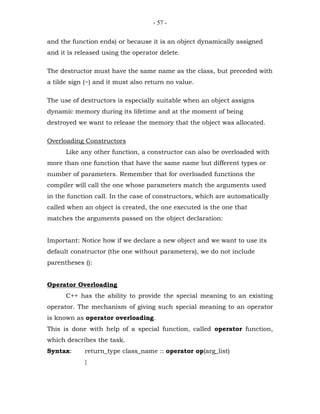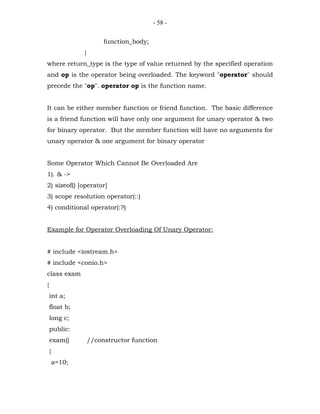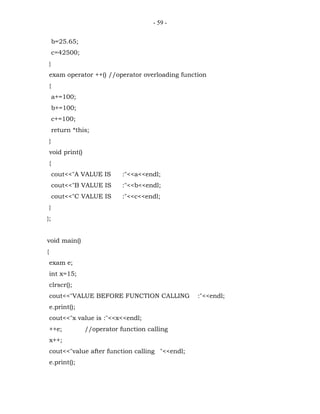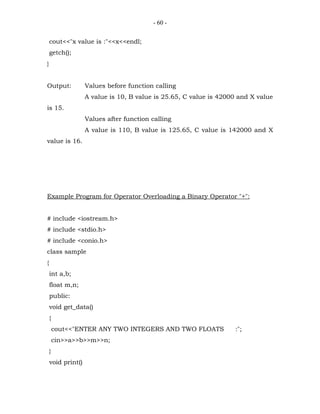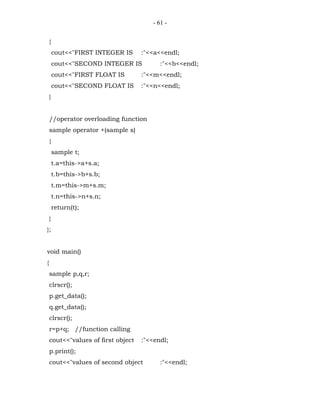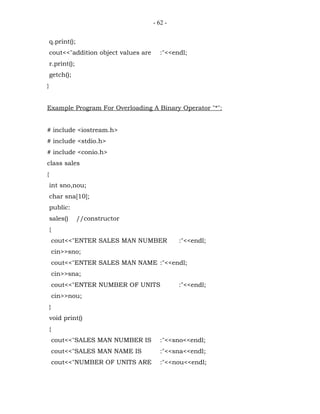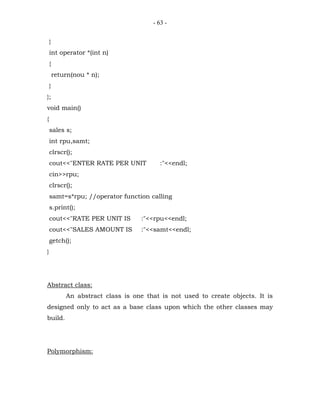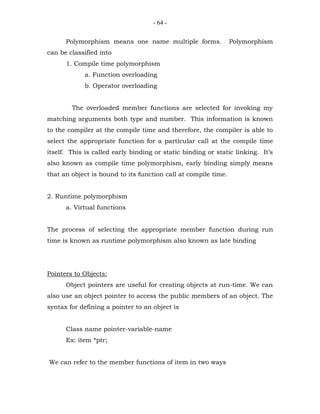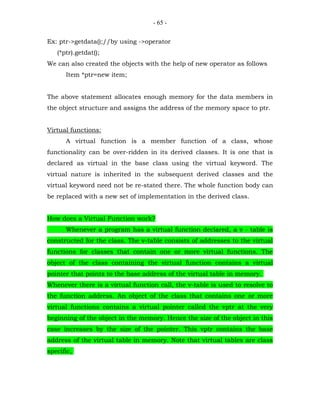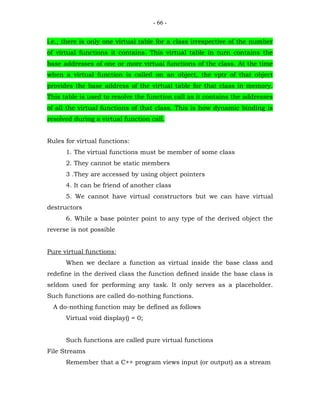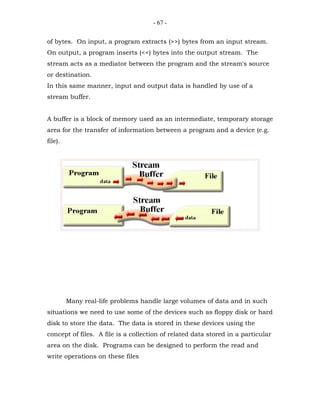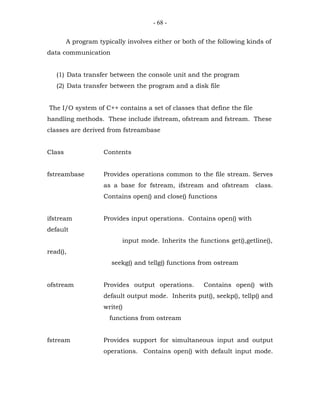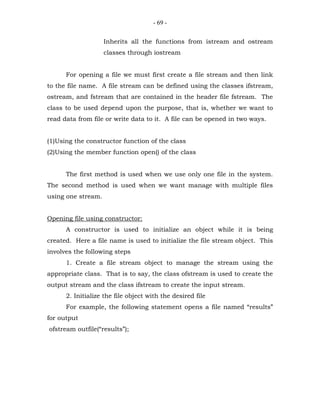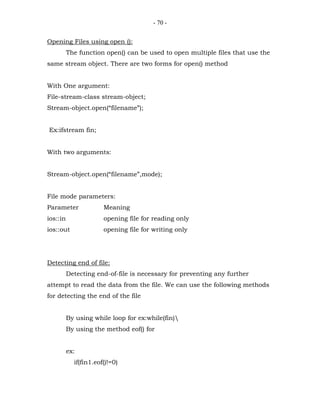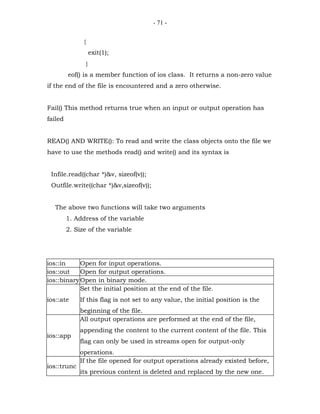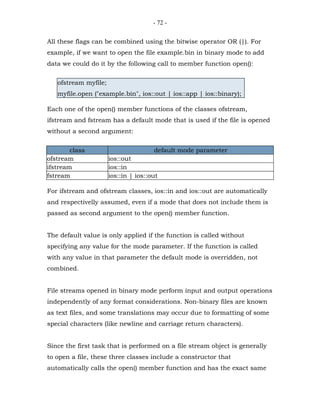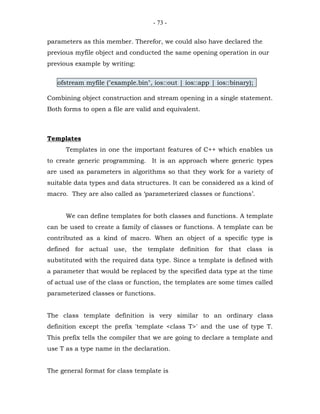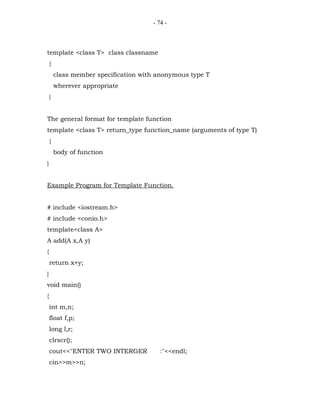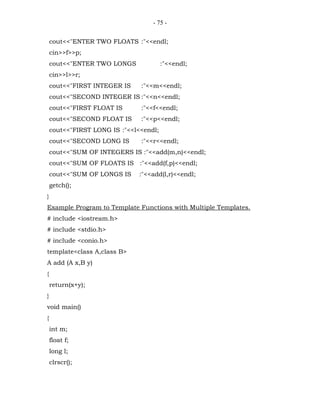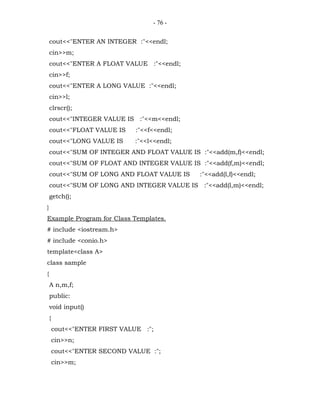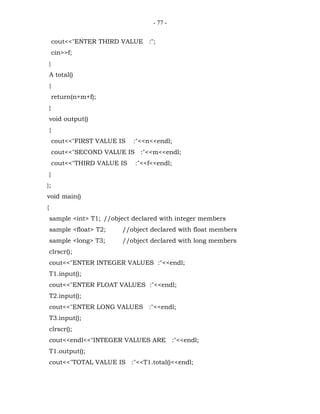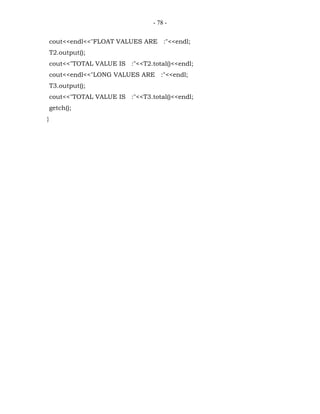Próximos SlideShares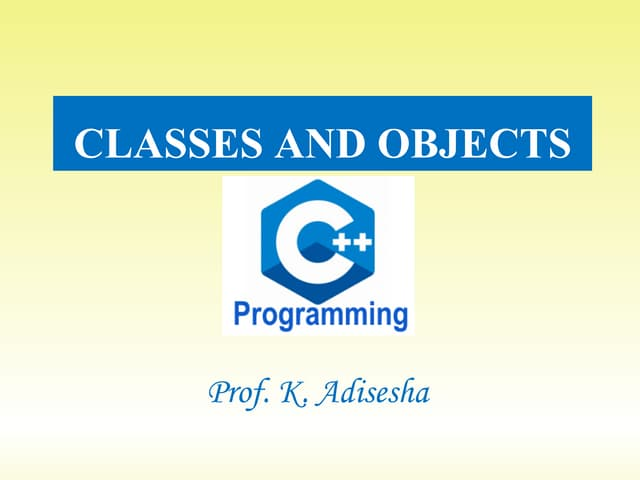Class and object
Carregando em ... 3
1 de 78
Anúncio

### My c++

1. -1- C++ (C with Classes) Object Oriented Programming (OOP) The term ‘‘Object Oriented Programming” means a programming language that fully supports the object oriented (features) style of programming. Introduction C++ is an object-oriented programming language developed by Bjarne Stroustrup at AT & T Bell Labs in the early 1980’s. Since the class concept was the major addition to the original C language, it is called as "C with classes". However later in 1983, the name was changed to C++. The idea of C++ comes from the 'C' increment operator "++", there by suggesting that C++ is an incremented version of 'C'. C++ is a superset of C. Therefore almost all C programs are valid C++ programs. Difference between C & C++ ‘C’ is a procedure-oriented programming language where everything is placed in the form of procedures or functions. While we concentrate on functions very little attention is given to the data that are being used by various functions & any function can access the information provided in other functions. It doesn’t model the real world problems very well. It employs top-down approach in program design. Where as ‘C++’ is an object-oriented programming language which treats data as a critical element in the program and it doesn’t allow moving freely around the system. It binds the data & the functions that operate on data together and protects it from accidental use of other
2. -2- functions. OOP allows us to decompose a problem into a number of entities called objects and built data and functions around these objects. Advantages of OOP 1. Object Oriented Approach 2. Closer to real world object 3. Dynamic Declaration 4. Data Security 5. Code Re-Usability & Extensibility Basic concepts of ‘Object-Oriented’ programming language Class A class is a blueprint or prototype that defines the variables and the methods common to all objects of a certain kind. A class is a logical construct of an object. A class is an abstract representation of something, whereas an object is a usable example of the class. A class is an expanded concept of a structure in C, instead of holding only data; it can hold both data and functions. Once a class has been defined, you can create any number of objects based on that class. Object An object is an instance (example or illustration) of a class. In terms of variables, a class would be the data type and an object would be the variable. An object is a physical reality. An object is a software bundle of related variables and methods. Software objects are often used to model real-world objects you find in everyday life.
3. -3- Polymorphism (many forms) An ability to have more than one function with the same name, each having different functionality. The function going to execute is determined at run time. Polymorphism refers to the fact that a single operation can have different behavior in different objects. E.g.: Overloading, Overriding. Overloading: Overloading is the practice of supplying more than one definition for a given function name in the same scope. The compiler is left to pick the appropriate version of the function or operator based on the arguments with which it is called. Inheritance The mechanism of creating new classes by deriving the features from existing classes is called Inheritance. In OOP the concept of inheritance provides the idea of reusability & extensibility. This means that we can add additional features of an existing class without modifying it. This is possible by deriving a new class from the existing one. The new class will have the combined features of both the classes. Encapsulation The method of packing all the data members & member functions into a single unit called class and the process is called ‘Encapsulation’ Data Hiding The process of hiding the data members and member functions from access by the other class objects other than the same class objects.
4. -4- The data is not accessible to the outside world and only those functions which are placed in the class can access it, these functions provide the interface between objects data and the program. The insulation of data from direct access by the program is called data hiding. Data Abstraction It is the process of accessing the data members & member functions with the objects. Abstraction refers to the act of representing essential features without including the background details and explanations. Classes use the concept of abstraction and defined as a list of abstraction attributes. Since the classes use the concept of data abstraction they are known as Abstract Data Type (ADT). Data Binding Binding refers to the act of associating an object or a class with its member. If we can call a method fn() on an object o of a class c, we say that the object o is binded with the method fn(). This happens at compile time and is known as static or compile - time binding. The calls to the virtual member functions are resolved during run-time. This mechanism is known as dynamic binding. The most prominent reason why a virtual function will be used is to have a different functionality in the derived class. The difference between a non-virtual member function and a virtual member function is the non-virtual member functions are resolved at compile time. Structure of C++ program: Include files Class declaration
5. -5- Member functions definition Main function { Local declaration; Object declaration; Executable coding; } Syntax for Class Declaration class class_name { access_specifier_1: data members; access_specifier_2: member functions; ………. } object_names; where class_name is a valid identifier for the class, object_names is an optional list of names for objects of this class. The body of the declaration can contain members, which can be either data or function declarations, or optionally access specifiers. All is very similar to the declaration on structures, except the new thing called access specifier. An access specifier is one of the following three keywords: private, public or protected. These specifiers modify the access rights that the members following them acquire:
6. -6- • private members of a class are accessible only within the class and by the public members of the same class or from their friends. • protected members are accessible from members of their same class and from their friends, but also from members of their derived classes. • public members are accessible from anywhere in the program where the object is visible. By default, all members of a class declared with the class keyword have private access for all its members. Function Definitions outside the class Member functions that are declared inside a class have to be defined separately outside the class. They should have a function definition outside the class as return_type class_name :: function_name(arguments list) { function body; } The membership label class_name :: tells the compiler that the function belongs to the class class_name. That is the scope of the function is restricted to the class_name specified in the header line. The symbol "::" is called Scope Resolution operator. The scope operator (::) specifies the class to which the member being declared belongs, granting exactly the same scope properties as if this function definition was directly included within the class definition. The only difference between defining a class member function completely within its class and to include only the prototype and later its definition,
7. -7- is that in the first case the function will automatically be considered an inline member function by the compiler, while in the second it will be a normal (not-inline) class member function, which in fact supposes no difference in behavior. Inline Function They are shortcut functions that are not actually called; rather their code is expanded at the point of execution. If a function is declared with the keyword inline, the compiler does not call the actual function instead it copies the code from the inline function directly into the calling function. Inline is a hint to the compiler that you would like the function to be inlined. The compiler is free to ignore the hint and make a real function call. this pointer: "this" is a pointer that points the address of the current object. The unique pointer is automatically passed to a member function when it is called. The pointer "this" acts as an implicit argument to all the member functions. Object Arrays We know that an array can be of any data type including struct, similarly we can also have arrays of variables that are of the type class. Such variables are called arrays of objects. class employ { char name; int age; public:
8. -8- void inputdata(); void outputdata(); }; ex:- employ emp; Create A Class With The Name Bank And Create An Array With 5 Cells And Accept Data And Print Data. Example for an Object Arrays. # include <stdio.h> # include <iostream.h> # include <conio.h> class bank { int acno,cbal; char cname; public: void input(); void output(); }; void bank::input() { cout<<"ENTER A/C NUMBER :"; cin>>acno; cout<<"ENTER CUSTOMER NAME :"; cin>>cname; cout<<"ENTER CURRENT BALANCE :";
9. -9- cin>>cbal; } void bank::output() { cout<<"ACCOUNT NUMBER IS :"<<acno<<endl; cout<<"CUSTOMER NAME IS :"<<cname<<endl; cout<<"CURRENT BALANCE IS :"<<cbal<<endl; } void main() { int i; bank b; for(i=0;i<5;i++) { cout<<"ENTER VALUES FOR CELL :"<<i<<endl; b[i].input(); } clrscr(); for(i=0;i<5;i++) { cout<<"THE VALUES OF THE CELL :"<<i<<endl; b[i].output(); getch(); } } Stream A stream is defined as a collection of pre-defined objects. The iostream (Input/Output stream) defines the following two objects. cout
10. - 10 - cout is an object, defined in standard output stream(ostream). The standard output stream normally flows to the screen. cout is an object of the class ostream_withassign, which is derived from ostream class. << The operator << is called insertion or put to operator. It directs the contents of the variable on its right to the object on its left. Screen <----- cout <----- << <--- variable e.g; screen<---cout<<"welcome"; cin cin is an object, defined in standard input stream (istream). This stream represents data coming from the keyboard. cin is an object of istream_withassign class, which is derived from istream class. >> This operator is called extraction or get from operator. It takes the value from the stream on its left and places it in the variable on its right. Keyboard ---> cin ---> >> ---> variable eg: keyboard-->cin>>x; Basic Data Types Built-in-Data types: int, char, float, double, void User-defined types: structure, union, class, enumeration Derived types: array, function, pointer, reference Reference Variable
11. - 11 - A reference variable provides an alias (alternative name) for a previously defined variable. Syntax: data_type &ref_var_name = variable; E.g.: int x = 200; int &xref = x; A reference variable must be initialized at the time of declaration. This establishes the correspondence between the reference and the data object that it names. Here the "&" is not an address operator. The notation datatype followed by "&" means that it is the reference to that datatype. Example for Reference Variable #include <stdio.h> #include <conio.h> # include <iostream.h> void main() { int a=200; int &b=a; int &c=b; clrscr(); cout<<"A VALUE IS :"<<a<<endl; cout<<"B VALUE IS :"<<b<<endl; cout<<"C VALUE IS :"<<c<<endl; getch(); b=55; cout<<"A VALUE IS :"<<a<<endl; cout<<"B VALUE IS :"<<b<<endl; cout<<"C VALUE IS :"<<c<<endl;
12. - 12 - getch(); c=3500; cout<<"A VALUE IS :"<<a<<endl; cout<<"B VALUE IS :"<<b<<endl; cout<<"C VALUE IS :"<<c<<endl; getch(); } Default Arguments C++ allows calling a function without specifying all its arguments. In such cases the function assigns a default value to the parameter which does not have a matching argument in the function call. We have to specify the default values when the function is declared. The compiler looks at the prototype (declaration) to see how many arguments the function uses and alerts the program for possible default values. One important point is that only the trailing arguments can have default values i.e. you must add default from right to left. It is not possible to provide a default value to a particular argument in the middle of the argument list. Ex: int add (int x, int y=0, int z=0); int mul (int a=1,int b=20,int c=40) Ex: add (10,20,30); add (10,20); add (10); add (); does not allow because there is no default value for argument x. Another Example for Default arguments
13. - 13 - # include <iostream.h> # include <stdio.h> # include <conio.h> int add(int a,int b,int c=0,int d=0,int e=0) { return (a+b+c+d+e); } void main() { int m,n,p,q,r; clrscr(); cout<<"ENTER ANY FIVE INTEGERS :"<<endl; cin>>m>>n>>p>>q>>r; cout<<"ADDITION OF 2 NUMBERS ARE :"<<add(m,n)<<endl; cout<<"ADDITION OF 3 NUMBERS ARE :"<<add(m,n,p)<<endl; cout<<"ADDITION OF 4 NUMBERS ARE :"<<add(m,n,p,q)<<endl; cout<<"ADDITION OF 5 NUMBERS ARE :"<<add(m,n,p,q,r)<<endl; getch(); } Memory Management Operators (Dynamic Memory Allocation) The part of the computer memory available to programmers, is divided into two areas: The Stack and The Heap (or Free Store). The Stack is used for those items that have a definite size and lifetime, for example, variables, arrays, etc. All the elements of a program where they are explicitly defined before compilation are stored ‘statically’.
14. - 14 - The Heap represents the remaining unused memory. This part of memory is generally used during the execution of the program. Any object that is created at run time is a dynamic object. Such an object has an unknown size and lifetime. Creating dynamic objects is very useful in creating highly interactive programs. Memory Management operators: C uses malloc() and calloc() functions to allocate memory dynamically at runtime. Similarly it uses the function free() to free dynamically allocated memory. Although C++ supports these functions, it also defines two unary operators 'new' and 'delete' that perform the task of allocating and freeing the memory in a better and easier way. Since these operators manipulate memory on the free store, these are also known as free store operators. To allocate the memory space dynamically during run time we can use ‘new’ operator & in order to free the memory space which is allocated with ‘new’ we can use the operator ‘delete’. The 'new' operator can be used to create objects of any type. Syntax: pointer_variable_name = new datatype Here pointer_variable is a pointer of type datatype. The new operator allocates sufficient memory to hold a data objects of TYPE datatype and return the address of the object. The datatype may be any valid datatype. The pointer variable holds the address of the memory space allocated. E.g. int *p;
15. - 15 - float *q; p = new int; q = new float ; Where p is a pointer of type int and q is a pointer of type float. When a data object is no longer needed, it is destroyed to release the memory space for reuse, in that case we can use 'delete' operator. Syntax: delete pointer_variable; The pointer_variable is the pointer that points to a data object created with new operator. E.g. delete p; delete []p//for freeing an array of size delete q; sales s; sales *q; q =&s; qinput() or sales.input(); qprint() or sales.print(); Example Program for Dynamic Memory Allocation. # include <iostream.h> # include <stdio.h> # include <conio.h> void main() { int n,*a,*p; clrscr();
16. - 16 - cout<<"ENTER NUMBER OF CELLS"; cin>>n; a=new int[n]; for(p=a;(p-a)<n;p++) { cout<<"ENTER ANY VALUE TO ARRAY :"; cin>>*p; } clrscr(); for(p=a;(p-a)<n;p++) { printf("THE CELL NUMBER IS %dt:",(p-a)); printf("THE CELL ADDRESS IS n:",p); cout<<"VALUE OF ARRAY :"<<*p<<endl; } delete a; getch(); } Example Program for Object Arrays with Dynamic Memory Allocation. # include <iostream.h> # include <stdio.h> # include <conio.h> class st { int sno, m1, m2, m3; char sna; public: void accept()
17. - 17 - { cout<<"ENTER STUDENT NUMBER:"; cin>>sno; cout<<"ENTER STUDENT'S NAME:"; cin>>sna; cout<<"ENTER THE MARKS OF THREE SUBJECTS:"; cin>>m1>>m2>>m3; } int get_tot() { return(m1+m2+m3); } void print() { cout<<"STUDENT NUMBER IS:"<<sno<<endl; cout<<"STUDENT'S NAME IS:"<<sna<<endl; cout<<"MARKS IN THREE SUBJECTS ARE:"<<m1<<"t"<<m2<<"t"<<m3<<endl; } }; void main() { st *p,*p1; int tot,av,n; clrscr(); cout<<"ENTER NUMBER OF CELLS:"; cin>>n; p=new st[n]; for(p1=p;(p1-p)<n;p1++) p1->accept();
18. - 18 - clrscr(); cout<<"THE VALUE OF THE ARRAY IS :"; for(p1=p;(p1-p)<n;p1++) { tot=p1->get_tot(); av=tot/3; printf("THE ADDRESS OF CELL IS n",p1); cout<<"THE VALUE OF CELL IS :"<<(p1-p)<<endl; p1->print(); cout<<"TOTAL MARKS ARE :"<<tot<<endl; cout<<"AVERAGE MARKS ARE :"<<av<<endl; getch(); } delete p; } Function Overloading 'Function overloading' means we have to perform the different operations by using the same method. The compiler will come to know the execution of a particular function depending on the maching of number of arguments & their return type during the function call Ex: int sum(int) int sum(int,int) void sum(float, int) void sum(float, int,int) In the above example we are using the same method 'sum' but the first method 'sum' performing it's operation by taking only one argument
19. - 19 - of 'type' & the second method 'sum' performing its operation by taking two arguments of type 'int' and so on. Ex:sum(15) //will make a call to first function sum sum(25,60)//will make a call to second function sum STATIC MEMBERS If we want to declare the data members that is common to all the objects created in that class we have to declare those data members as the static data members & the syntax for declaring static data member is static data-type variable-name; Ex: static int a; The above data member 'a' will be shared by all the objects created in that class i.e only one copy of that data member is created for all the object without creating a separate copy. Whenever we create a data member as a static it will be initialized to '0' automatically, and we can define the value for the static data members outside the class i.e with the help of the scope resolution operator & it's syntax is data-type class-name :: data-member-name=value Ex: int example :: a; It means the value of a is initialized to 0 int example :: a=10; It means the value of a is initialized to 10 Static member function: We can also declare a member function as a static. The syntax for declaring a member function as static is Syntax: static return-type function-name(arguments); Inheritance:
20. - 20 - The process of creating a new class by deriving the features from existing classes. It provides the concept of reusability & extensibility. The term 'reusability' means the ability to use the data provided in one class by another class & 'Extensibility' provides the ability to add some more data members & member functions to the already existing class The main class is called as 'base class' & the class which is acquiring the features of the base class is called as derived class & the syntax for defining the derived class is class derived_class_name : access_specifier base_class_name { //data members & member function of derived class }
21. - 21 - The 'access-specifer' specifies the method of accessing the information provided in the base class & it will be accessed in any one of the three ways. 1. private 2. protected 3. public Inheriting in private mode: If the derived class acquires the features of base class in private mode base derived private will become private protected '' private public private Inheriting in protected mode: If we acquire the features of base class in 'protected mode' its characteristics will be base derived private will become private protected " protected public " protected Inheriting in public mode: In 'public' access specifier the following the characteristics of the base class in derived class base derived private will become private protected " private public " public
22. - 22 - In all the above three cases the 'private' data members of the base class is not inheritable. If we want to access those 'private' data members we have to declare a function in 'public' mode in the base class & with that member function we can access them in the derived class If we want to define those data members in the derived class itself we have declare those data members in 'protected' mode, but these will be available up to one level only i.e it's accessible to the immediate class which is accessing it's features Both 'private' & 'protected' data members are not accessible in the main() function. 'public' data-members & member functions will be accessible anywhere in our program i.e they are accessible in the main() function also Reusability is another important feature of OOP. It is always nice if we could reuse something that already exists rather than trying to create the same all over again. C++ strongly supports the concept of reusability. The C++ classes can be reused in several ways. Once a class has been written and tested, it can be adopted by other programmers to send their requirements. Creating new classes, reusing the properties of the existing ones basically does this. The mechanism of deriving a new class from an old one is called "Inheritance" (or derivation). The old class referred to as the base class and the new one is called derived class. The derived class inherits some or all the properties of the base class. A class can also inherit properties from more than one class of from more than one level. A derived class with only one base class is called single inheritance, and one derived class with several base classes is called
23. - 23 - multiple inheritance. On the other hand, the properties of one class may be inherited by more than one class. This process is known as hierarchical inheritance. The mechanism of deriving a class from another derived class is known as multilevel inheritance. The combination of any of two inheritances is called hybrid inheritance. Defining a Derived Class A derived class is defined by specifying it's relationship with the base class in addition to it's own details. Syntax: class derived class_name : visibility_mode base class name { - ------- - ------- //members of derived class - ------- }; The colon (:) indicates that the derived class name is derived from the base class name. The visibility mode is optional and if present, it may be either private or public. The default visibility mode is private. This mode specifies whether the features of the base class are privately derived or publicly derived. When a base class is privately inherited by a derived class, ‘public members’ of the base class become ‘private members’ of the derived class and therefore the public members of the base class can only be accessed by the member functions of the derived class. They are inaccessible to the objects of the derived class. A public member of a class can be accessed by its own objects using the dot operator. The result is that no member of the base class is accessible to the objects of the derived class. When a base class is publicly inherited by a derived class, public members of the base class becomes the pubic members to the derived
24. - 24 - class and therefore they are accessible to the objects of the dedrived class. In both the cases private members are not inherited and therefore the private members of a base class will never become the members of it's derived class. E.g.: class B:public A { - - - - - - //members of class B ------ ------ ------ }; Example Program for Single Inheritance. # include <iostream.h> # include <conio.h> class a { int x,y; void input(int p,int r) { x=p; y=r; } void print() { cout<<"X VALUE IS :"<<x<<endl; cout<<"Y VALUE IS :"<<y<<endl;
25. - 25 - } class b:public a //deriving a derived class { int m,n; public: void accept() { cout<<"ENTER TWO INTEGERS :"; cin>>m,n; } void display() { cout<<"M VALUE IS :"<<m<<endl; cout<<"N VALUE IS :"<<n<<endl; } }; void main() { b t; //object declared for class b clrscr(); t.input(253,445);//member function of base class t.accept(); //function of the derived class clrscr(); t.print(); //function of the base class t.display(); //function of the derived class getch(); } Example Program for Multilevel Inheritance or a Derived Class from another Derived Class.
26. - 26 - # include <iostream.h> # include <stdio.h> # include <string.h> # include <conio.h> class student { int sno; char sna,sadd; public: void input() { cout<<"ENTER STUDENT NUMBER :"; cin>>sno; cout<<"ENTER STUDENT'S NAME :"; cin>>sna; cout<<"ENTER STUDENT'S ADDRESS :"; cin>>sadd; } void output() { cout<<"STUDENT NUMBER IS "<<sno<<endl; cout<<"STUENT'S NAME IS "<<sna<<endl; cout<<"STUDENT'S ADDRESS IS "<<sadd<<endl; } }; class fees:public student { int tf,fp,due; char cou;
27. - 27 - public: void accept() { cout<<"ENTER COURSE NAME :"; cin>>cou; cout<<"ENTER TOTAL FEE :"; cin>>tf; cout<<"HOW MUCH FEE IS PAID :"; cin>>fp; due=tf-fp; } void display() { cout<<"COURSE NAME IS :"<<cou<<endl; cout<<"TOTAL FEE IS :"<<tf<<endl; cout<<"FEE PAID IS :"<<fp<<endl; cout<<"DUE FEE IS :"<<tf-fp<<endl; } }; class marks:public fees { int tm,mm,mp,mc,am; char res; public: void marksin() { cout<<"ENTER MARKS IN MATHS :"; cin>>mm; cout<<"ENTER MARKS IN PHYSICS :"; cin>>mp;
28. - 28 - cout<<"ENTER MARKS IN CHEMISTRY :"; cin>>mc; } void resout() { tm=mm+mp+mc; am=tm/3; if(am>75) strcpy(res,"DISTINCTION"); else if(am>=60) strcpy(res,"FIRST CLASS"); else if(am>=50) strcpy(res,"SECOND CLASS"); else if(am>=35) strcpy(res,"THIRD CLASS"); else strcpy(res,"FAILED"); cout<<"MARKS IN MATHS ARE :"<<mm<<endl; cout<<"MARKS IN PHYSICS ARE :"<<mp<<endl; cout<<"MARKS IN CHEMISTRY ARE :"<<mc<<endl; cout<<"TOTAL MARKS ARE :"<<tm<<endl; cout<<"AVERAGE MARKS ARE :"<<am<<endl; cout<<"RESULT IS--------------:"<<res<<endl; } }; void main() { clrscr(); marks obj; //object declaration
29. - 29 - obj.input();//member function of class student obj.accept();//member function of class fees obj.marksin();//member function of class marks clrscr(); obj.output(); //member function of class student obj.display();//member function of class fees obj.resout();//member function of class marks getch(); } Multiple Inheritance: A class can inherit the attributes of two or more classes. This is known as multiple inheritance. Multiple inheritance allows combining the features of several existing classes as a starting point for defining a new class. Syntax: class derived_class : visibility label base class, visibility label base class……… { -- - - - - - - - - - ----------- ----------- ----------- } Where visibility label is either public or private. Comma separate the base classes. Protected Members:
30. - 30 - C++ provides a third visibility modifier protected, which serve a limited purpose in inheritance. A member declared as protected is accessible by the member functions with in its class and any class derived from it as the public member of the base class. It cannot be accessed by the functions outside these classes. When a protected member is inherited in public mode, it becomes protected in the derived class too and therefore it is accessible by the member functions of the derived class. It is also ready for further inheritance. A protected member is inherited in the private mode derivation, that becomes private in the derived class. Although it is available to the member functions of the derived class. It is not available for further inheritance since private members cannot be inherited. E.g.: class A { protected: int a; ------- ------- ------- }; class B:public A { - - - - - - //members of class A are - - - - - - //protected to class B ------ };
31. - 31 - class C:private B { - - - - - - //members of class A are - - - - - - //private to class C ------ }; class D:public C { - - - - - - //members of class A cannot be - - - - - - //accessed in class D ------ }; Example Program For Multiple Inheritance And Protected Members. (Two Or More Base Classes One Derived Class Is Called Multiple Inheritance). # include <iostream.h> # include <conio.h> class employ { protected: int eno,bs; char ena,eadd; public: void input() { cout<<"ENTER EMPLOY NUMBER :"; cin>>eno; cout<<"ENTER EMPLOY NAME :"; cin>>ena;
32. - 32 - cout<<"ENTER EMPLOY ADDRESS :"; cin>>eadd; cout<<"ENTER BASIC SALARY :"; cin>>bs; } }; class allow { protected: int da,hra,cca; public: void accept() { cout<<"ENTER DA:"; cin>>da; cout<<"ENTER HRA:"; cin>>hra; cout<<"ENTER CCA:"; cin>>cca; } }; class ded { protected: int pf,it; public: void dedinput() { cout<<"ENTER PROVIDENT FUND:"; cin>>pf;
33. - 33 - cout<<"ENTER INCOME TAX:"; cin>>it; } }; class salary:public employ,public allow,public ded { int tall,tded,gs,ns; public: void calc_sal() { tall=da+hra+cca; tded=pf+it; gs=bs+tall; ns=gs-tded; } void output() { cout<<"EMPLOY NUMBER IS:"<<eno<<endl; cout<<"EMPLOY NAME IS :"<<ena<<endl; cout<<"EMPLOY ADDRESS IS :"<<eadd<<endl; cout<<"BASIC SALARY IS :"<<bs<<endl; cout<<"EMPLOY'S DA IS :"<<da<<endl; cout<<"EMPLOY'S HRA IS :"<<hra<<endl; cout<<"EMPLOY'S CCA IS :"<<cca<<endl; cout<<"EMPLOY'S PF IS :"<<pf<<endl; cout<<"EMPLOY'S IT IS :"<<it<<endl; cout<<"TOTAL ALLOWANCES :"<<tall<<endl; cout<<"TOTAL DEDUCTIONS :"<<tded<<endl; cout<<"EMPLOY'S GROSS SALARY IS :"<<gs<<endl; cout<<"EMPLOY'S NET SALARY IS :"<<ns<<endl;
34. - 34 - } }; void main() { salary s; s.input(); s.accept(); s.dedinput(); clrscr(); s.calc_sal(); s.output(); getch(); } Hierarchical Inheritance: In the hierarchical inheritance different classes are derived from one base class. A subclass can be constructed by inheriting the properties of the base class. A subclass can serve as a base class for the lower classes and so on. Example Program for Hierarchical Inheritance. # include <iostream.h> # include <conio.h> # include<string.h> class student { int sno; char sna,sadd; public:
35. - 35 - void input() { cout<<"ENTER STUDENT'S NUMBER :"; cin>>sno; cout<<"ENTER STUDENT'S NAME :"; cin>>sna; cout<<"ENTER STUDET'S ADDRESS :"; cin>>sadd; } void output() { cout<<"STUDENT'S NUMBER IS :"<<sno<<endl; cout<<"STUDENT'S NAME IS :"<<sna<<endl; cout<<"STUDENT'S ADDRESS IS :"<<sadd<<endl; } }; class lang { int mt,me; public: void accept() { cout<<"ENTER MARKS IN TELUGU:"; cin>>mt; cout<<"ENTER MARKS IN ENGLISH :"; cin>>me; } void display() { cout<<"TELUGU MARKS ARE :"<<mt<<endl;
36. - 36 - cout<<"ENGLISH MARKS ARE :"<<me<<endl; } }; class mathes:public lang { int mm,mp,mc; public: void sentry() { cout<<"ENTER MARKS IN MATHS :"; cin>>mm; cout<<"ENTER MARKS IN PHYSICS :"; cin>>mp; cout<<"ENTER MARKS IN CHEMISTRY:"; cin>>mc; } void sprint() { cout<<"MARKS IN MATHS ARE :"<<mm<<endl; cout<<"MARKS IN PHYSICS ARE :"<<mp<<endl; cout<<"MARKS IN CHEMISTRY ARE :"<<mc<<endl; cout<<"TOTAL MARKS ARE :"<<mt+me+mm+mp+mc<<endl; } }; class computers:public lang { int mmt,mph,mcs; public: void sentry() {
37. - 37 - cout<<"ENTER MARKS IN MATHS :"; cin>>mmt; cout<<"ENTER MARKS IN PHYSICS :"; cin>>mph; cout<<"ENTER MARKS IN COMPUTERS:"; cin>>mcs; } void sprint() { cout<<"MARKS IN MATHS ARE :"<<mmt<<endl; cout<<"MARKS IN PHYSICS ARE :"<<mph<<endl; cout<<"MARKS IN COMPTERS ARE :"<<mcs<<endl; cout<<"TOTAL MARKS ARE :"<<mt+me+mmt+mph+mcs<<endl; } }; class arts:public lang { int mhs,mec,mci; public: void sentry() { cout<<"ENTER MARKS IN HISTORY :"; cin>>mhs; cout<<"ENTER MARKS IN ECONOMICS:"; cin>>mec; cout<<"ENTER MARKS IN CIVICS :"; cin>>mci; } void sprint()
38. - 38 - { cout<<"HISTORY MARKS ARE :"<<mhs<<endl; cout<<"ECONOMICS MARKS ARE :"<<mec<<endl; cout<<"CIVICS MARKS ARE :"<<mci<<endl; cout<<"TOTAL MARKS ARE :"<<mt+me+mhs+mec+mci<<endl; } }; void main() { mathes m; computers cs; arts a; clrscr(); m.accept(); m.sentry(); clrscr(); cs.accept(); cs.sentry(); clrscr(); a.accept(); a.sentry(); clrscr(); m.display(); m.sprint(); getch(); cs.display(); cs.sprint(); getch(); a.display(); a.sprint();
39. - 39 - getch(); } Example for Hybrid Example. (The Combination Of Any Two Inheritance Is Called Hybrid Inheritance. # include <iostream.h> # include <stdio.h> # include <conio.h> # include <string.h> class student { protected: int sno; char sna,sadd; public: void input() { cout<<"ENTER STUENT NUMBER :"; cin>>sno; cout<<"ENTER STUDENT'S NAME :"; cin>>sna; cout<<"ENTER STUDENT'S ADDRESS:"; cin>>sadd; } }; class test:public student { protected: int mm,mp,mc;
40. - 40 - public: void mentry() { cout<<"ENTER MARKS IN MATHS :"; cin>>mm; cout<<"ENTER MARKS IN PHYSICS :"; cin>>mp; cout<<"ENTER MARKS IN CHEMISTRY:"; cin>>mc; } }; class sports { protected: int sm; public: void sentry() { cout<<"ENTER SPORTS MARKS :"; cin>>sm; } }; class result:public test, public sports { int tot,avg; char res; public: void findres() { tot=mm+mp+mc;
41. - 41 - avg=tot/3; if(sm>50) avg=avg+5; if(avg>=75) strcpy(res,"DISTINCTION"); else if(avg>=60) strcpy(res,"FIRST CLASS"); else if(avg>=50) strcpy(res,"SECOND CLASS"); else if(avg>=35) strcpy(res,"THIRD CLASS"); else if(avg<35) strcpy(res,"FAILED"); } void display() { cout<<"STUDENT NUMBER IS :"<<sno<<endl; cout<<"STUDENT'S NAME IS :"<<sna<<endl; cout<<"STUDENT'S ADDRESS IS :"<<sadd<<endl; cout<<"MARKS IN MATHS ARE :"<<mm<<endl; cout<<"MARKS IN PHYSICS ARE :"<<mp<<endl; cout<<"MARKS IN CHEMISTRY ARE:"<<mc<<endl; cout<<"TOTAL MARKS ARE :"<<tot<<endl; cout<<"AVERAGE MARKS ARE :"<<avg<<endl; cout<<"RESULT OBTAINED :"<<res<<endl; } }; void main() { result r;
42. - 42 - clrscr(); r.input(); //member function of student class r.mentry(); //member function of test class r.sentry(); //member function of sports class r.findres(); //member function of result class clrscr(); r.display(); getch(); } Friend function A friend function is a special function which is not a member of any class but it is declared to access the private member data & functions provided in that class. C++ allows a common function to be made friendly with one or more classes, there by allowing the function to have access to the private data of these classes, such a function need not be a member of any of these classes, only thing is to declare that function as a friend to the class. Syntax for defining a friend function class sample { private: member declaration; public: member function declaration; friend return_type function_name(arguments); //friend };
43. - 43 - The function declaration should be preceded by the keyword "friend". The function definition does not use the keyword friend or the scope resolution operator "::". The functions that are declared with the key word friend are known as friend functions. A function can be declared as a friend in any number of classes. A friend function should possess the following characteristics (1)It is not in the scope of the class to which it has been declared as friend (2)It cannot be called with the scope resolution operator (3)It can be invoked just like a normal function without help of any object (4)It can be declared either in public or private without affecting its meaning (5)Usually it has the objects as arguments (6)It cannot access the member names directly and has to use an object name and dot membership operator with each member name (Ex. s.a); Friend Function Example. # include <iostream.h> # include <conio.h> class sample; class myclass { int a; public: void input() { cout<<"ENTER VALUE FOR A:"; cin>>a;
44. - 44 - } void output() { cout<<"A VALUE IS :"<<a<<endl; } friend int getsum(myclass m,sample a) }; class sample { int x; public: void accept() { cout<<"ENTER A VALUE :"; cin>>x; } //friend function declaration friend int getsum(myclass m,sample s) }; int getsum(myclass m,sample s) { return(m.a+s.x); } void main() { myclass c; sample d; int t; clrscr(); c.input();
45. - 45 - d.accept(); t=getsum(c,d); //friend function calling clrscr(); c.output(); d.accept(); cout<<"TOTAL VALUE IS :"<<t<<endl; getch(); } 2nd Example for a Friend Function. # include <iostream.h> # include <stdio.h> # include <conio.h> class allow; class ded; class employ { int eno,bs; char ena; public: void accept() { cout<<"ENTER EMPLOY NUMBER :"; cin>>eno; cout<<"ENTER EMPLOY NAME :"; cin>>ena; cout<<"ENTER BASIC SALARY :"; cin>>bs; }
46. - 46 - friend void calc_sal(employ e,allow a,ded d); }; class allow { int da,hra,cca; public: void getallow() { cout<<"ENTER DA, HRA, CCA :"<<endl; cin>>da>>hra>>cca; } friend void calc_sal(employ e,allow a,ded d); }; class ded { int pf,it; public: void getded() { cout<<"ENTER PF, IT :"<<endl; cin>>pf>>it; } friend void calc_sal(employ e,allow a,ded d); }; void calc_sal(employ e,allow a,ded d) { int gs,ns,tall,tded; tall=d.pf+d.it+a.cca; tded=d.pf+d.it; gs=e.bs+tall;
47. - 47 - ns=gs-tded; clrscr(); cout<<"ENTER EMPLOY NUMBER :"<<e.eno<<endl; cout<<"ENTER EMPLOY NAME :"<<e.ena<<endl; cout<<"ENTER BASIC SALARY :"<<e.bs<<endl; cout<<"DA IS :"<<a.da<<endl; cout<<"HRA IS :"<<a.hra<<endl; cout<<"CCA IS :"<<a.cca<<endl; cout<<"PF IS :"<<d.pf<<endl; cout<<"IT IS :"<<d.it<<endl; cout<<"GROSS SALARY IS :"<<gs<<endl; cout<<"NET SALARY IS :"<<ns<<endl; } void main() { employ emp; allow all; ded s; clrscr(); emp.accept(); all.getallow(); s.getded(); calc_sal(emp,all,s); getch(); } Friend Functions with Objects as Arguments and Return Type. # include <iostream.h> # include <conio.h>
48. - 48 - class test; class sample { int a; float b; public: void input() { cout<<"ENTER ANY INTEGER AND FLOAT VALUES :"<<endl; cin>>a>>b; } void display() { cout<<"A VALUE IS :"<<a<<endl; cout<<"B VALUE IS :"<<b<<endl; } friend sample setsum(sample x,test y); }; class test { int m; float n; public: void accept() { cout<<"ENTER INTEGER AND FLOAT :"<<endl; cin>>m>>n; } void print() {
49. - 49 - cout<<"M VALUE IS :"<<m<<endl; cout<<"N VALUE IS :"<<n<<endl; } friend sample setsum(sample x,test y); }; sample setsum(sample x,test y) { sample r; r.a=x.a+y.m; r.b=x.b+y.n; return r; } void main() { sample s,p; test t; clrscr(); s.input(); t.accept(); clrscr(); p=setsum(s,t); cout<<"VALUE OF FIRST OBJECT IS :"<<endl; s.display(); cout<<"VALUE OF SECOND OBJECT IS :"<<endl; t.print(); cout<<"VALUE OF RESULT OBJECT IS :"<<endl; p.display(); getch(); }
50. - 50 - Constructors A constructor is a special member function whose task is to initialize the objects or variables of a class. The constructor is invoked implicitly (automatically) whenever an object is created. It is called constructor, because it will be called implicitly upon the construction of an object. A constructor is declared as follows. class class_name { data members; public: class_name(arguments) //constructor { statements; } }; E.g.: class sample { private: int a,b; float x,y; public: sample() //constructor function { a=100; b=200; x=35.15; y=564.25;
51. - 51 - } }; void main() { clrscr(); sample s; getch(); } Characteristics of a constructor 1. It should have the same name as the class name 2. They are invoked automatically when the objects are created. 3. They cannot be called explicitly. 4. Like C++ functions they can have default arguments. 5. They do not return any value not even void 6. They cannot be inherited but the derived class can call the base class constructor 7. It cannot be virtual 8. We cannot refer to their addresses 9. They will make implicit called to new & delete operator when memory allocation is required Types of constructors (3 Types) 1. Default constructor (Non Parameterized): It won’t take any arguments. 2. Parameterized constructor: It can take any no. of arguments. 3. Copy constructor: It will take a reference of an object as an argument. Example for Constructors # include <iostream.h>
52. - 52 - # include <stdio.h> # include <conio.h> class test { int a; float b; long c; public: test() //constructor function { a=230; b=654.13; c=54865; } void output() { cout<<"INTEGER VALUE IS :"<<a<<endl; cout<<"FLOAT VALUE IS :"<<b<<endl; cout<<"LONG VALUE IS :"<<c<<endl; } }; void main() { clrscr(); test t,s,r; clrscr(); cout<<"VALUES OF T ARE :"<<endl; t.output(); cout<<"VALUES OF S ARE :"<<endl;
53. - 53 - s.output(); cout<<"VALUES OF R ARE :"<<endl; r.output(); getch(); } Example for Constructor Function. # include <iostream.h> # include <conio.h> class stud { int sno,tf,fp,due; char sna,cou; public: stud() { cout<<"enter student's number :"; cin>>sno; cout<<"enter student's name :"; cin>>sna; cout<<"enter course name :"; cin>>cou; cout<<"enter total fee is :"; cin>>tf; cout<<"enter fee paid :"; cin>>fp; due=tf-fp;
54. - 54 - } void output() { cout<<"student's number is :"<<sno<<endl; cout<<"student's name is :"<<sna<<endl; cout<<"course name is :"<<cou<<endl; cout<<"total name is :"<<tf<<endl; cout<<"fee paid is :"<<fp<<endl; cout<<"due fee is :"<<due<<endl; } }; void main() { clrscr(); stud s,t,u; clrscr(); cout<<"values of s"<<endl; s.output(); cout<<"values of t"<<endl; t.output(); cout<<"values of u"<<endl; u.output(); getch(); } Example for Constructor with Arguments. # include <iostream.h>
55. - 55 - # include <conio.h> # include <string.h> class sales { int sno,samt; char sna; public: sales(int a,char n[],int b) { sno=a; strcpy(sna,n); samt=b; } void output() { cout<<"SALES MAN NUMBER IS :"<<sno<<endl; cout<<"SALES MAN NAME IS :"<<sna<<endl; cout<<"SALES AMOUNT IS :"<<samt<<endl; } }; void main() { clrscr(); sales t(100,"RAVI",2500); sales b=sales(101,"KIRAN",3000); cout<<"VALUES OF T :"<<endl; t.output(); cout<<"VALUES OF B :"<<endl; b.output(); getch();
56. - 56 - } Constructors in derived class: If the base class contain a constructor with one or more arguments, then it is mandatory for the derived class to have a constructor and to pass the arguments to the bas class constructors, since while applying inheritance we usually create the objects by using the derived class. Thus, it makes sense for the derived class to pass arguments to the base class constructor. When both the base class & derived class contain constructors, the base constructor is executed first and then the constructor in the derived class is executed. The syntax for defining the constructor in the derived class is Constructor(arglist) : initialization-section { assignment section } Destructor A destructor as the name implies, is used to destroy the objects that have been created by a constructor. Like a constructor, the destructor is also a member function whose name is same as the class name and is preceded by a tilde (~) symbol. A destructor neither takes any arguments nor returns any value. It will be invoked implicitly by the compiler upon exit from the program to clean up the storage that is no longer accessible. The destructor fulfills the opposite functionality. It is automatically called when an object is destroyed, either because its scope of existence has finished (for example, if it was defined as a local object within a function
57. - 57 - and the function ends) or because it is an object dynamically assigned and it is released using the operator delete. The destructor must have the same name as the class, but preceded with a tilde sign (~) and it must also return no value. The use of destructors is especially suitable when an object assigns dynamic memory during its lifetime and at the moment of being destroyed we want to release the memory that the object was allocated. Overloading Constructors Like any other function, a constructor can also be overloaded with more than one function that have the same name but different types or number of parameters. Remember that for overloaded functions the compiler will call the one whose parameters match the arguments used in the function call. In the case of constructors, which are automatically called when an object is created, the one executed is the one that matches the arguments passed on the object declaration: Important: Notice how if we declare a new object and we want to use its default constructor (the one without parameters), we do not include parentheses (): Operator Overloading C++ has the ability to provide the special meaning to an existing operator. The mechanism of giving such special meaning to an operator is known as operator overloading. This is done with help of a special function, called operator function, which describes the task. Syntax: return_type class_name :: operator op(arg_list) {
58. - 58 - function_body; } where return_type is the type of value returned by the specified operation and op is the operator being overloaded. The keyword "operator" should precede the "op". operator op is the function name. It can be either member function or friend function. The basic difference is a friend function will have only one argument for unary operator & two for binary operator. But the member function will have no arguments for unary operator & one argument for binary operator Some Operator Which Cannot Be Overloaded Are 1). & -> 2) sizeof() [operator] 3) scope resolution operator(::) 4) conditional operator(:?) Example for Operator Overloading Of Unary Operator: # include <iostream.h> # include <conio.h> class exam { int a; float b; long c; public: exam() //constructor function { a=10;
59. - 59 - b=25.65; c=42500; } exam operator ++() //operator overloading function { a+=100; b+=100; c+=100; return *this; } void print() { cout<<"A VALUE IS :"<<a<<endl; cout<<"B VALUE IS :"<<b<<endl; cout<<"C VALUE IS :"<<c<<endl; } }; void main() { exam e; int x=15; clrscr(); cout<<"VALUE BEFORE FUNCTION CALLING :"<<endl; e.print(); cout<<"x value is :"<<x<<endl; ++e; //operator function calling x++; cout<<"value after function calling "<<endl; e.print();
60. - 60 - cout<<"x value is :"<<x<<endl; getch(); } Output: Values before function calling A value is 10, B value is 25.65, C value is 42000 and X value is 15. Values after function calling A value is 110, B value is 125.65, C value is 142000 and X value is 16. Example Program for Operator Overloading a Binary Operator "+": # include <iostream.h> # include <stdio.h> # include <conio.h> class sample { int a,b; float m,n; public: void get_data() { cout<<"ENTER ANY TWO INTEGERS AND TWO FLOATS :"; cin>>a>>b>>m>>n; } void print()
61. - 61 - { cout<<"FIRST INTEGER IS :"<<a<<endl; cout<<"SECOND INTEGER IS :"<<b<<endl; cout<<"FIRST FLOAT IS :"<<m<<endl; cout<<"SECOND FLOAT IS :"<<n<<endl; } //operator overloading function sample operator +(sample s) { sample t; t.a=this->a+s.a; t.b=this->b+s.b; t.m=this->m+s.m; t.n=this->n+s.n; return(t); } }; void main() { sample p,q,r; clrscr(); p.get_data(); q.get_data(); clrscr(); r=p+q; //function calling cout<<"values of first object :"<<endl; p.print(); cout<<"values of second object :"<<endl;
62. - 62 - q.print(); cout<<"addition object values are :"<<endl; r.print(); getch(); } Example Program For Overloading A Binary Operator "*": # include <iostream.h> # include <stdio.h> # include <conio.h> class sales { int sno,nou; char sna; public: sales() //constructor { cout<<"ENTER SALES MAN NUMBER :"<<endl; cin>>sno; cout<<"ENTER SALES MAN NAME :"<<endl; cin>>sna; cout<<"ENTER NUMBER OF UNITS :"<<endl; cin>>nou; } void print() { cout<<"SALES MAN NUMBER IS :"<<sno<<endl; cout<<"SALES MAN NAME IS :"<<sna<<endl; cout<<"NUMBER OF UNITS ARE :"<<nou<<endl;
63. - 63 - } int operator *(int n) { return(nou * n); } }; void main() { sales s; int rpu,samt; clrscr(); cout<<"ENTER RATE PER UNIT :"<<endl; cin>>rpu; clrscr(); samt=s*rpu; //operator function calling s.print(); cout<<"RATE PER UNIT IS :"<<rpu<<endl; cout<<"SALES AMOUNT IS :"<<samt<<endl; getch(); } Abstract class: An abstract class is one that is not used to create objects. It is designed only to act as a base class upon which the other classes may build. Polymorphism:
64. - 64 - Polymorphism means one name multiple forms. Polymorphism can be classified into 1. Compile time polymorphism a. Function overloading b. Operator overloading The overloaded member functions are selected for invoking my matching arguments both type and number. This information is known to the compiler at the compile time and therefore, the compiler is able to select the appropriate function for a particular call at the compile time itself. This is called early binding or static binding or static linking. It’s also known as compile time polymorphism, early binding simply means that an object is bound to its function call at compile time. 2. Runtime polymorphism a. Virtual functions The process of selecting the appropriate member function during run time is known as runtime polymorphism also known as late binding Pointers to Objects: Object pointers are useful for creating objects at run-time. We can also use an object pointer to access the public members of an object. The syntax for defining a pointer to an object is Class name pointer-variable-name Ex: item *ptr; We can refer to the member functions of item in two ways
65. - 65 - Ex: ptr->getdata();//by using ->operator (*ptr).getdat(); We can also created the objects with the help of new operator as follows Item *ptr=new item; The above statement allocates enough memory for the data members in the object structure and assigns the address of the memory space to ptr. Virtual functions: A virtual function is a member function of a class, whose functionality can be over-ridden in its derived classes. It is one that is declared as virtual in the base class using the virtual keyword. The virtual nature is inherited in the subsequent derived classes and the virtual keyword need not be re-stated there. The whole function body can be replaced with a new set of implementation in the derived class. How does a Virtual Function work? Whenever a program has a virtual function declared, a v - table is constructed for the class. The v-table consists of addresses to the virtual functions for classes that contain one or more virtual functions. The object of the class containing the virtual function contains a virtual pointer that points to the base address of the virtual table in memory. Whenever there is a virtual function call, the v-table is used to resolve to the function address. An object of the class that contains one or more virtual functions contains a virtual pointer called the vptr at the very beginning of the object in the memory. Hence the size of the object in this case increases by the size of the pointer. This vptr contains the base address of the virtual table in memory. Note that virtual tables are class specific,
66. - 66 - i.e., there is only one virtual table for a class irrespective of the number of virtual functions it contains. This virtual table in turn contains the base addresses of one or more virtual functions of the class. At the time when a virtual function is called on an object, the vptr of that object provides the base address of the virtual table for that class in memory. This table is used to resolve the function call as it contains the addresses of all the virtual functions of that class. This is how dynamic binding is resolved during a virtual function call. Rules for virtual functions: 1. The virtual functions must be member of some class 2. They cannot be static members 3 .They are accessed by using object pointers 4. It can be friend of another class 5. We cannot have virtual constructors but we can have virtual destructors 6. While a base pointer point to any type of the derived object the reverse is not possible Pure virtual functions: When we declare a function as virtual inside the base class and redefine in the derived class the function defined inside the base class is seldom used for performing any task. It only serves as a placeholder. Such functions are called do-nothing functions. A do-nothing function may be defined as follows Virtual void display() = 0; Such functions are called pure virtual functions File Streams Remember that a C++ program views input (or output) as a stream
67. - 67 - of bytes. On input, a program extracts (>>) bytes from an input stream. On output, a program inserts (<<) bytes into the output stream. The stream acts as a mediator between the program and the stream's source or destination. In this same manner, input and output data is handled by use of a stream buffer. A buffer is a block of memory used as an intermediate, temporary storage area for the transfer of information between a program and a device (e.g. file). Many real-life problems handle large volumes of data and in such situations we need to use some of the devices such as floppy disk or hard disk to store the data. The data is stored in these devices using the concept of files. A file is a collection of related data stored in a particular area on the disk. Programs can be designed to perform the read and write operations on these files
68. - 68 - A program typically involves either or both of the following kinds of data communication (1) Data transfer between the console unit and the program (2) Data transfer between the program and a disk file The I/O system of C++ contains a set of classes that define the file handling methods. These include ifstream, ofstream and fstream. These classes are derived from fstreambase Class Contents fstreambase Provides operations common to the file stream. Serves as a base for fstream, ifstream and ofstream class. Contains open() and close() functions ifstream Provides input operations. Contains open() with default input mode. Inherits the functions get(),getline(), read(), seekg() and tellg() functions from ostream ofstream Provides output operations. Contains open() with default output mode. Inherits put(), seekp(), tellp() and write() functions from ostream fstream Provides support for simultaneous input and output operations. Contains open() with default input mode.
69. - 69 - Inherits all the functions from istream and ostream classes through iostream For opening a file we must first create a file stream and then link to the file name. A file stream can be defined using the classes ifstream, ostream, and fstream that are contained in the header file fstream. The class to be used depend upon the purpose, that is, whether we want to read data from file or write data to it. A file can be opened in two ways. (1)Using the constructor function of the class (2)Using the member function open() of the class The first method is used when we use only one file in the system. The second method is used when we want manage with multiple files using one stream. Opening file using constructor: A constructor is used to initialize an object while it is being created. Here a file name is used to initialize the file stream object. This involves the following steps 1. Create a file stream object to manage the stream using the appropriate class. That is to say, the class ofstream is used to create the output stream and the class ifstream to create the input stream. 2. Initialize the file object with the desired file For example, the following statement opens a file named “results” for output ofstream outfile(“results”);
70. - 70 - Opening Files using open (): The function open() can be used to open multiple files that use the same stream object. There are two forms for open() method With One argument: File-stream-class stream-object; Stream-object.open(“filename”); Ex:ifstream fin; With two arguments: Stream-object.open(“filename”,mode); File mode parameters: Parameter Meaning ios::in opening file for reading only ios::out opening file for writing only Detecting end of file: Detecting end-of-file is necessary for preventing any further attempt to read the data from the file. We can use the following methods for detecting the end of the file By using while loop for ex:while(fin) By using the method eof() for ex: if(fin1.eof()!=0)
71. - 71 - { exit(1); } eof() is a member function of ios class. It returns a non-zero value if the end of the file is encountered and a zero otherwise. Fail() This method returns true when an input or output operation has failed READ() AND WRITE(): To read and write the class objects onto the file we have to use the methods read() and write() and its syntax is Infile.read((char *)&v, sizeof(v)); Outfile.write((char *)&v,sizeof(v)); The above two functions will take two arguments 1. Address of the variable 2. Size of the variable ios::in Open for input operations. ios::out Open for output operations. ios::binary Open in binary mode. Set the initial position at the end of the file. ios::ate If this flag is not set to any value, the initial position is the beginning of the file. All output operations are performed at the end of the file, appending the content to the current content of the file. This ios::app flag can only be used in streams open for output-only operations. If the file opened for output operations already existed before, ios::trunc its previous content is deleted and replaced by the new one.
72. - 72 - All these flags can be combined using the bitwise operator OR (|). For example, if we want to open the file example.bin in binary mode to add data we could do it by the following call to member function open(): ofstream myfile; myfile.open ("example.bin", ios::out | ios::app | ios::binary); Each one of the open() member functions of the classes ofstream, ifstream and fstream has a default mode that is used if the file is opened without a second argument: class default mode parameter ofstream ios::out ifstream ios::in fstream ios::in | ios::out For ifstream and ofstream classes, ios::in and ios::out are automatically and respectivelly assumed, even if a mode that does not include them is passed as second argument to the open() member function. The default value is only applied if the function is called without specifying any value for the mode parameter. If the function is called with any value in that parameter the default mode is overridden, not combined. File streams opened in binary mode perform input and output operations independently of any format considerations. Non-binary files are known as text files, and some translations may occur due to formatting of some special characters (like newline and carriage return characters). Since the first task that is performed on a file stream object is generally to open a file, these three classes include a constructor that automatically calls the open() member function and has the exact same
73. - 73 - parameters as this member. Therefor, we could also have declared the previous myfile object and conducted the same opening operation in our previous example by writing: ofstream myfile ("example.bin", ios::out | ios::app | ios::binary); Combining object construction and stream opening in a single statement. Both forms to open a file are valid and equivalent. Templates Templates in one the important features of C++ which enables us to create generic programming. It is an approach where generic types are used as parameters in algorithms so that they work for a variety of suitable data types and data structures. It can be considered as a kind of macro. They are also called as ‘parameterized classes or functions’. We can define templates for both classes and functions. A template can be used to create a family of classes or functions. A template can be contributed as a kind of macro. When an object of a specific type is defined for actual use, the template definition for that class is substituted with the required data type. Since a template is defined with a parameter that would be replaced by the specified data type at the time of actual use of the class or function, the templates are some times called parameterized classes or functions. The class template definition is very similar to an ordinary class definition except the prefix 'template <class T>' and the use of type T. This prefix tells the compiler that we are going to declare a template and use T as a type name in the declaration. The general format for class template is
74. - 74 - template <class T> class classname { class member specification with anonymous type T wherever appropriate } The general format for template function template <class T> return_type function_name (arguments of type T) { body of function } Example Program for Template Function. # include <iostream.h> # include <conio.h> template<class A> A add(A x,A y) { return x+y; } void main() { int m,n; float f,p; long l,r; clrscr(); cout<<"ENTER TWO INTERGER :"<<endl; cin>>m>>n;
75. - 75 - cout<<"ENTER TWO FLOATS :"<<endl; cin>>f>>p; cout<<"ENTER TWO LONGS :"<<endl; cin>>l>>r; cout<<"FIRST INTEGER IS :"<<m<<endl; cout<<"SECOND INTEGER IS :"<<n<<endl; cout<<"FIRST FLOAT IS :"<<f<<endl; cout<<"SECOND FLOAT IS :"<<p<<endl; cout<<"FIRST LONG IS :"<<l<<endl; cout<<"SECOND LONG IS :"<<r<<endl; cout<<"SUM OF INTEGERS IS :"<<add(m,n)<<endl; cout<<"SUM OF FLOATS IS :"<<add(f,p)<<endl; cout<<"SUM OF LONGS IS :"<<add(l,r)<<endl; getch(); } Example Program to Template Functions with Multiple Templates. # include <iostream.h> # include <stdio.h> # include <conio.h> template<class A,class B> A add (A x,B y) { return(x+y); } void main() { int m; float f; long l; clrscr();
76. - 76 - cout<<"ENTER AN INTEGER :"<<endl; cin>>m; cout<<"ENTER A FLOAT VALUE :"<<endl; cin>>f; cout<<"ENTER A LONG VALUE :"<<endl; cin>>l; clrscr(); cout<<"INTEGER VALUE IS :"<<m<<endl; cout<<"FLOAT VALUE IS :"<<f<<endl; cout<<"LONG VALUE IS :"<<l<<endl; cout<<"SUM OF INTEGER AND FLOAT VALUE IS :"<<add(m,f)<<endl; cout<<"SUM OF FLOAT AND INTEGER VALUE IS :"<<add(f,m)<<endl; cout<<"SUM OF LONG AND FLOAT VALUE IS :"<<add(l,f)<<endl; cout<<"SUM OF LONG AND INTEGER VALUE IS :"<<add(l,m)<<endl; getch(); } Example Program for Class Templates. # include <iostream.h> # include <conio.h> template<class A> class sample { A n,m,f; public: void input() { cout<<"ENTER FIRST VALUE :"; cin>>n; cout<<"ENTER SECOND VALUE :"; cin>>m;
77. - 77 - cout<<"ENTER THIRD VALUE :"; cin>>f; } A total() { return(n+m+f); } void output() { cout<<"FIRST VALUE IS :"<<n<<endl; cout<<"SECOND VALUE IS :"<<m<<endl; cout<<"THIRD VALUE IS :"<<f<<endl; } }; void main() { sample <int> T1; //object declared with integer members sample <float> T2; //object declared with float members sample <long> T3; //object declared with long members clrscr(); cout<<"ENTER INTEGER VALUES :"<<endl; T1.input(); cout<<"ENTER FLOAT VALUES :"<<endl; T2.input(); cout<<"ENTER LONG VALUES :"<<endl; T3.input(); clrscr(); cout<<endl<<"INTEGER VALUES ARE :"<<endl; T1.output(); cout<<"TOTAL VALUE IS :"<<T1.total()<<endl;
78. - 78 - cout<<endl<<"FLOAT VALUES ARE :"<<endl; T2.output(); cout<<"TOTAL VALUE IS :"<<T2.total()<<endl; cout<<endl<<"LONG VALUES ARE :"<<endl; T3.output(); cout<<"TOTAL VALUE IS :"<<T3.total()<<endl; getch(); }
Anúncio